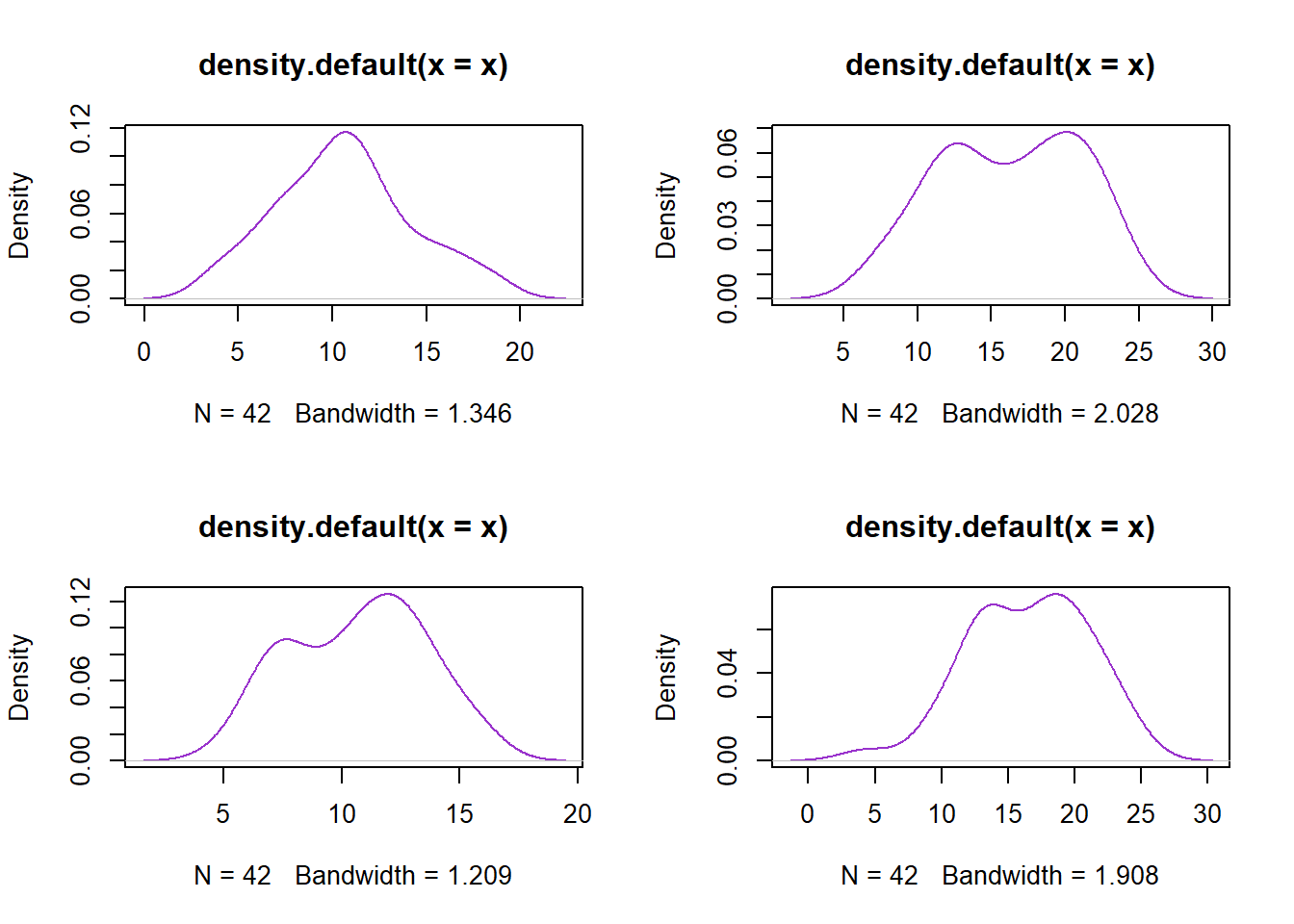## Open the data set

library(xlsx)
data <- read.xlsx("Experiment2.xlsx", sheetIndex = "Sheet1")

## Check the first and last six rows, check the data structure and generate a summary

head(data)
##   NA.       Pre     Post  Group
## 1   1 10.221375 19.27253 system
## 2   2 11.915686 18.81053 system
## 3   3 18.337294 22.71338 system
## 4   4 14.803235 16.46261 system
## 5   5  7.123360 18.42337 system
## 6   6  6.090328 20.80002 system
tail(data)
##    NA.      Pre     Post       Group
## 79  79 12.55211 13.88570 traditional
## 80  80 15.78812 14.64949 traditional
## 81  81 15.17438 23.67276 traditional
## 82  82 12.83547 22.57924 traditional
## 83  83 10.67936 11.44996 traditional
## 84  84 13.41753 21.68929 traditional
str(data)
## 'data.frame':    84 obs. of  4 variables:
##  $NA. : Factor w/ 84 levels "1","10","11",..: 1 12 23 34 45 56 67 78 84 2 ... ##$ Pre  : num  10.22 11.92 18.34 14.8 7.12 ...
##  $Post : num 19.3 18.8 22.7 16.5 18.4 ... ##$ Group: Factor w/ 2 levels "system","traditional": 1 1 1 1 1 1 1 1 1 1 ...
summary(data)
##       NA.          Pre              Post               Group
##  1      : 1   Min.   : 3.940   Min.   : 4.30   system     :42
##  10     : 1   1st Qu.: 7.916   1st Qu.:12.91   traditional:42
##  11     : 1   Median :10.728   Median :16.83
##  12     : 1   Mean   :10.647   Mean   :16.37
##  13     : 1   3rd Qu.:12.623   3rd Qu.:20.07
##  14     : 1   Max.   :18.354   Max.   :24.62
##  (Other):78

## Clean data by removing the irrelevant first column

data <- data[,-1]

## Subset data by test administration and group

SysPre <- data[data$Group == "system",]$Pre
SysPost <- data[data$Group == "system",]$Post
TradPre <- data[data$Group == "traditional",]$Pre
TradPost <- data[data$Group == "traditional",]$Post

## Conduct normality testing for the data for each group and test administration

library(nortest)
nortest <- apply(nordata, 2, function(x) ad.test(x))
nortest #Data sets tend to assume Normal Distribution
## $SysPre ## ## Anderson-Darling normality test ## ## data: x ## A = 0.24322, p-value = 0.7516 ## ## ##$SysPost
##
##  Anderson-Darling normality test
##
## data:  x
## A = 0.63055, p-value = 0.09373
##
##
## $TradPre ## ## Anderson-Darling normality test ## ## data: x ## A = 0.4832, p-value = 0.2178 ## ## ##$TradPost
##
##  Anderson-Darling normality test
##
## data:  x
## A = 0.32375, p-value = 0.5146

## Generate Probability Density Function Plots

par(mfrow=c(2,2))
apply(nordata, 2, function(x) plot(density(x), col = "darkorchid"))## NULL

## Generate Descriptive Statistics

library(psych)
describeBy(data$Pre, data$Group) #Mean is the measure for central tendency given the result of the normality test
##
##  Descriptive statistics by group
## group: system
##    vars  n  mean   sd median trimmed  mad  min   max range skew kurtosis
## X1    1 42 10.61 3.61  10.56   10.51 3.58 3.94 18.35 14.41 0.22    -0.46
##      se
## X1 0.56
## --------------------------------------------------------
##    vars  n  mean   sd median trimmed  mad  min   max range  skew kurtosis
## X1    1 42 10.69 2.84  11.23   10.66 3.06 5.19 15.84 10.65 -0.08    -1.07
##      se
## X1 0.44
describeBy(data$Post, data$Group)
##
##  Descriptive statistics by group
## group: system
##    vars  n  mean   sd median trimmed  mad  min  max range  skew kurtosis
## X1    1 42 16.23 4.76  16.35   16.37 6.19 7.43 23.9 16.47 -0.14    -1.23
##      se
## X1 0.73
## --------------------------------------------------------
##    vars  n mean   sd median trimmed  mad min   max range  skew kurtosis
## X1    1 42 16.5 4.48  17.01   16.65 5.34 4.3 24.62 20.32 -0.32    -0.35
##      se
## X1 0.69

## Conduct of test for significant differences in the Pretest of System and Traditional (Unpaired)

library(lawstat)
levene.test(data$Pre, data$Group, location = "mean") #Variances are equal; Student's t-test
##
##  classical Levene's test based on the absolute deviations from the
##  mean ( none not applied because the location is not set to median
##  )
##
## data:  data$Pre ## Test Statistic = 0.84335, p-value = 0.3611 t.test(SysPre, TradPre, var.equal = 1) # No significant difference in the pretest ratings between System and Traditional ## ## Two Sample t-test ## ## data: SysPre and TradPre ## t = -0.10981, df = 82, p-value = 0.9128 ## alternative hypothesis: true difference in means is not equal to 0 ## 95 percent confidence interval: ## -1.486689 1.331146 ## sample estimates: ## mean of x mean of y ## 10.60762 10.68539 ## Conduct of test for significant differences in the Pretest and PostTest for Group System (Paired) t.test(SysPost, SysPre, paired = 1) # There exists a significant difference; it seems that post test ratings tend to be higher than pre test ratings by about 3.88 to 7.37 at 95% confidence interval. ## ## Paired t-test ## ## data: SysPost and SysPre ## t = 6.5136, df = 41, p-value = 8.048e-08 ## alternative hypothesis: true difference in means is not equal to 0 ## 95 percent confidence interval: ## 3.880497 7.368109 ## sample estimates: ## mean of the differences ## 5.624303 ## Conduct of test for significant differences in the Pretest and PostTest for Group Traditional (Paired) t.test(TradPost, TradPre, paired = 1) # There exists a significant difference; it seems that post test ratings tend to be higher than pre test ratings by about 4.68 to 6.96 at 95% confidence interval. ## ## Paired t-test ## ## data: TradPost and TradPre ## t = 10.302, df = 41, p-value = 6.083e-13 ## alternative hypothesis: true difference in means is not equal to 0 ## 95 percent confidence interval: ## 4.675165 6.955091 ## sample estimates: ## mean of the differences ## 5.815128 ## Conduct of test for significant differences in the PostTest of System and Traditional (Unpaired) levene.test(data$Post, data$Group, location = "mean") #Variances are equal; Student's t-test ## ## classical Levene's test based on the absolute deviations from the ## mean ( none not applied because the location is not set to median ## ) ## ## data: data$Post
## Test Statistic = 0.64932, p-value = 0.4227
t.test(SysPost, TradPost, var.equal = 1) # No significant difference in the post test ratings between System and Traditional
##
##  Two Sample t-test
##
##  16.23193  16.50052